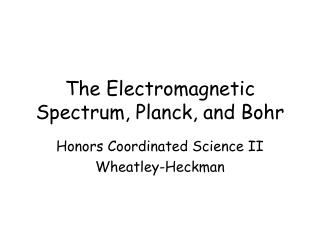DownloadDownload PresentationThe Electromagnetic Spectrum, Planck, and Bohr

# The Electromagnetic Spectrum, Planck, and Bohr

Télécharger la présentation## The Electromagnetic Spectrum, Planck, and Bohr

- - - - - - - - - - - - - - - - - - - - - - - - - - - E N D - - - - - - - - - - - - - - - - - - - - - - - - - - -
##### Presentation Transcript

1. The Electromagnetic Spectrum, Planck, and Bohr Honors Coordinated Science II Wheatley-Heckman

2. Electromagnetic Spectrum • Extends from low to high wavelengths.

3. Electromagnetic Wave Properties • λ (lambda) = wavelength • Distance between 2 corresponding points on adjacent waves. • ν (nu) = frequency • The number of waves that pass a point during a period of time. • Units = Hertz (Hz) = 1 wave/second

4. Constant Speed • All forms of EM radiation move at a constant speed of c = 3.00 x 108 m/s. • The relationship between frequency and wavelength can be expressed mathematically: c = λν

5. Practice Problem #1 • Calculate the frequency of a light wave with a wavelength of 515 nm. • Hint: You must first convert 515 nm to meters. • 515 nm = 515 x 10-9 m

6. Max Planck • Proposes that objects can only emit energy in small and specific amounts. • Planck discovers the following relationship: • E = energy (measured in Joules) • h = Planck's constant = 6.626 x10-34 J*s • ν = frequency E = h ν

7. 3 Useful Equations c = λν E = hν c h λ E =

8. More Practice Problems • Calculate the frequency of a wave with an Energy of 3.75 x 10-6 J. • A near infrared wave has a wavelength of 1.00 x 10-6 meters. Calculate the energy of this wave.

9. Flame Test Why do different elements emit a different color of light when exposed to a flame?

10. Line Spectra When the light from the flame is passed through a prism a spectrum is formed:

11. A spectrum that contains only certain colors (wavelengths) is called a line spectrum. When different elements are vaporized in the flame, they each produce a unique spectrum: this is a kind of “atomic fingerprint” that can be useful in identifying elements. But why does this occur? And where does this energy come from?

12. The Bohr Model Niels Bohr combines the ideas of: Rutherford (planetary model with a nucleus and circling electrons) Planck (quantized amounts of energy) Bohr concludes that the energy of the electrons in an atom has to be quantized. This means that electrons can only have certain amounts of energy.

13. An electron in the ground state is closest to the nucleus (n=1). When it absorbs energy, it enters an excited state and moves to a higher energy level.

14. Bohr’s Model of the H atom Energy is emitted when an electron falls from an excited state to ground state. The released energy causes the unique line spectrum. Bohr uses his model and Planck’s equation to calculate the frequencies for the line spectrum of hydrogen.

15. Limitations of the Bohr Model Bohr’s model is able to explain the line spectrum for hydrogen, but could not explain the spectra of more complex atoms that have more electrons.